# Example No. D4(a) Multifamily Dwelling

A multifamily dwelling has 40 dwelling units.

Meters in two banks of 20 each and individual feeders to each dwelling unit.

One-half of the dwelling units are equipped with electric ranges not exceeding 12 kW each. Assume range kW rating equivalent to kVA rating in accordance with 220.55. Other half of ranges are gas ranges.

Area of each dwelling unit is 840 square feet.

Laundry facilities on premises are available to all tenants. Add no circuit to individual dwelling unit.

Calculated Load for Each Dwelling Unit (See Article 220)

 General lighting: 840 sq. ft. at 3 volt-amperes per sq. ft. 2520 VA Special appliance load: electric range (see 250.55) 8000 VA

Minimum Number of Branch Circuits Required for Each Dwelling Unit [See 210.11(A)]:

General lighting load: 2520 volt-amperes ÷ 120 V = 21 amperes or two 15-ampere, 2-wire circuits; or two 20-ampere, 2-wire circuits.

Small appliance load: Two 2-wire circuits of 12 AWG wire [see 220.11(C)(1)].

Range circuit: 8000 volt-amperes ÷ 240 volts = 33 amperes or a circuit of two 8 AWG conductors and one 10 AWG conductor as per 210.19(A)(3).

Minimum Size Feeder Required for Each Dwelling Unit (See 215.2)

 Calculated load (Article 220) General lighting 2520 VA Small appliance (two 20-ampere circuits) 3000 VA Subtotal computed load (without ranges) 5520 VA

Application of Demand Factor (see Table 220.42)

 First 3000 volt-amperes at 100% 3000 VA 5520 VA - 3000 VA = 2520 volt-amperes at 35% 882 VA Net calculated load (without ranges) 3882 VA Range load 8000 VA Net calculated load (with ranges) 11,882 VA

Size of Each Feeder (See 215.2)

 For 120/240-volt, 3-wire system (without ranges):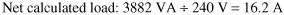= 16.2 A For 120/240-volt, 3-wire system (with ranges):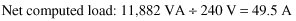= 49.5 A

Feeder Neutral

 Lighting and small appliance 3882 VA Range: 8000 volt-amperes at 70% (see Section 220.61) (only for apart- ments with electric ranges) 5600 VA Net calculated load (neutral) 9482 VA

9482 VA ÷ 240 V = 39.5 A

Minimum Size Feeders Required from Service Equipment to Meter Bank (for 20 Dwelling Units10 with Ranges)

 Total calculated load: Lighting and small appliance 20 x 5520 volt-amperes 110,400 VA Application of Demand Factor: First 3000 volt-amperes at 100% 3000 VA 110,400 VA - 3000 VA = 107,400 volt-amperes at 35% 37,950 VA Net calculated load 40,590 VA Range: 10 ranges (less than 12 kVA), (see Col. A, Table 220.55) 25,000 VA Net calculated load (with ranges) 65,590 VA

Net calculated load for 120/240-volt, 3-wire system: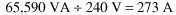Feeder Neutral

 Lighting and small appliance 40,590 VA Range: 25,000 volt-amperes at 70% [see 220.61(B)] 17,500 VA Net calculated load (neutral) 58,090 VA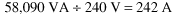Further Demand Factor [220.61(B)]:

 200 amperes at 100% 200 A 242A - 200 A = 42 amperes at 70% 29 A Net calculated load (neutral) 229 A

Minimum Size Main Feeder (or Service Conductors) Required (Less House Load) (for 40 Dwelling Units20 with Ranges)

 Total calculated load: Lighting and small appliance load, 40 x 5520 volt-amperes 220,800 VA

Application of Demand Factor (from Table 220.42)

 First 3000 volt-amperes at 100% 3000 VA Next 120,000 VA - 3000 VA = 117,000 volt-amperes at 35% 40,950 VA Remainder 220,800 VA - 120,000 VA = 100,800 volt-amperes at 25% 25,200 VA Net calculated load 69,150 VA Range load, 20 ranges (less than 12 kVA) (see Col. C, Table 220.55) 35,000 VA Net calculated load 104,150 VA For 120/240-volt, 3-wire system: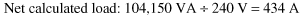Feeder Neutral

 Lighting and small appliance load 69,150 VA Range load, 35,000 volt-amperes at 70% [see 220.61(B)] 24,500 VA Calculated load (neutral) 93,650 VA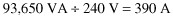Further Demand Factor [See 220.61(B)]

 200 amperes at 100% 200 A 390A - 200 A = 190 amperes at 70% 133 A Net calculated load (neutral) 333 A

[See Tables Table 310.16 through 310.21 and 310.15(B)(2) and (B)(4).]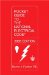Pocket Guide to the National Electrical Code(R), 2005 Edition (8th Edition)
ISBN: 0131480014
EAN: 2147483647
Year: 2004
Pages: 120